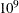# The OPTQP Procedure

### ODS Tables

PROC OPTQP creates three ODS (Output Delivery System) tables by default. The first table, ProblemSummary, is a summary of the input QP problem. The second table, SolutionSummary, is a brief summary of the solution status. The third table, PerformanceInfo, is a summary of performance options. You can use ODS table names to select tables and create output data sets. For more information about ODS, see the SAS Output Delivery System: User's Guide.

If you specify a value of 2 for the PRINTLEVEL= option, then the ProblemStatistics table is produced. This table contains information about the problem data. See the section Problem Statistics for more information.

If you specify the DETAILS option in the PERFORMANCE statement, then the Timing table is produced.

Table 12.3 lists all the ODS tables that can be produced by the OPTQP procedure, along with the statement and option specifications required to produce each table.

Table 12.3: ODS Tables Produced by PROC OPTQP

ODS Table Name

Description

Statement

Option

ProblemSummary

Summary of the input QP problem

PROC OPTQP

PRINTLEVEL=1 (default)

SolutionSummary

Summary of the solution status

PROC OPTQP

PRINTLEVEL=1 (default)

ProblemStatistics

Description of input problem data

PROC OPTQP

PRINTLEVEL=2

PerformanceInfo

List of performance options and their values

PROC OPTQP

PRINTLEVEL=1 (default)

Timing

Detailed solution timing

PERFORMANCE

DETAILS

A typical output of PROC OPTQP is shown in Figure 12.5.

Figure 12.5: Typical OPTQP Output

The OPTQP Procedure

Performance Information
Execution Mode Single-Machine

Problem Summary
Problem Name BANDM
Objective Sense Minimization
Objective Function ....1
RHS ZZZZ0001

Number of Variables 472
Bounded Above 0
Bounded Below 472
Bounded Above and Below 0
Free 0
Fixed 0

Number of Constraints 305
LE (<=) 0
EQ (=) 305
GE (>=) 0
Range 0

Constraint Coefficients 2494

Hessian Diagonal Elements 25
Hessian Elements Above the Diagonal 16

Solution Summary
Solver QP
Algorithm Interior Point
Objective Function ....1
Solution Status Optimal
Objective Value 16352.342037

Primal Infeasibility 1.127493E-12
Dual Infeasibility 1.684333E-12
Bound Infeasibility 4.520378E-16
Duality Gap 1.25305E-12
Complementarity 1.2956352E-8

Iterations 23
Presolve Time 0.00
Solution Time 0.23

You can create output data sets from these tables by using the ODS OUTPUT statement. This can be useful, for example, when you want to create a report to summarize multiple PROC OPTQP runs. The output data sets that correspond to the preceding output are shown in Figure 12.6, where you can also find (in the row following the heading of each data set in the display) the variable names that are used in the table definition (template) of each table.

Figure 12.6: ODS Output Data Sets

 Problem Summary

Obs Label1 cValue1 nValue1
1 Problem Name BANDM .
2 Objective Sense Minimization .
3 Objective Function ....1 .
4 RHS ZZZZ0001 .
5     .
6 Number of Variables 472 472.000000
7 Bounded Above 0 0
8 Bounded Below 472 472.000000
9 Bounded Above and Below 0 0
10 Free 0 0
11 Fixed 0 0
12     .
13 Number of Constraints 305 305.000000
14 LE (<=) 0 0
15 EQ (=) 305 305.000000
16 GE (>=) 0 0
17 Range 0 0
18     .
19 Constraint Coefficients 2494 2494.000000
20     .
21 Hessian Diagonal Elements 25 25.000000
22 Hessian Elements Above the Diagonal 16 16.000000

 Solution Summary

Obs Label1 cValue1 nValue1
1 Solver QP .
2 Algorithm Interior Point .
3 Objective Function ....1 .
4 Solution Status Optimal .
5 Objective Value 16352.342037 16352
6     .
7 Primal Infeasibility 1.127493E-12 1.127493E-12
8 Dual Infeasibility 1.684333E-12 1.684333E-12
9 Bound Infeasibility 4.520378E-16 4.520378E-16
10 Duality Gap 1.25305E-12 1.25305E-12
11 Complementarity 1.2956352E-8 1.2956352E-8
12     .
13 Iterations 23 23.000000
14 Presolve Time 0.00 0
15 Solution Time 0.23 0.230999

#### Problem Statistics

Optimizers can encounter difficulty when solving poorly formulated models. Information about data magnitude provides a simple gauge to determine how well a model is formulated. For example, a model whose constraint matrix contains one very large entry (on the order of) can cause difficulty when the remaining entries are single-digit numbers. The PRINTLEVEL=2 option in the OPTQP procedure causes the ODS table ProblemStatistics to be generated. This table provides basic data magnitude information that enables you to improve the formulation of your models.

The example output in Figure 12.7 demonstrates the contents of the ODS table ProblemStatistics.

Figure 12.7: ODS Table ProblemStatistics

The OPTQP Procedure

Problem Statistics
Number of Constraint Matrix Nonzeros 4
Maximum Constraint Matrix Coefficient 2
Minimum Constraint Matrix Coefficient 1
Average Constraint Matrix Coefficient 1.25

Number of Linear Objective Nonzeros 2
Maximum Linear Objective Coefficient 3
Minimum Linear Objective Coefficient 2
Average Linear Objective Coefficient 2.5

Number of Lower Triangular Hessian Nonzeros 1
Number of Diagonal Hessian Nonzeros 2
Maximum Hessian Coefficient 20
Minimum Hessian Coefficient 2
Average Hessian Coefficient 6.75

Number of RHS Nonzeros 2
Maximum RHS 100
Minimum RHS 1
Average RHS 50.5

Maximum Number of Nonzeros per Column 2
Minimum Number of Nonzeros per Column 2
Average Number of Nonzeros per Column 2

Maximum Number of Nonzeros per Row 2
Minimum Number of Nonzeros per Row 2
Average Number of Nonzeros per Row 2